# More properties of the definite integral

Here we will look at some properties of the Riemann integral that are not directly related to its evaluation, but are more of a theoretical interest.

Theorem.
Let f be a Riemann integrable function on [a,b]. Then the equalities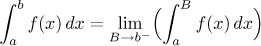and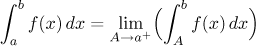are true.

Note that the integrals in limits make sense. Consider the first equality: We assume that f is Riemann integrable on [a,b]. If we pick some B between a and b (note that in the limit we approach b from the left, now we can see why), then by the Theorem here part (i), f is also Riemann integrable on [a,B] and so we can integrate inside the limit. The situation is shown in the following picture.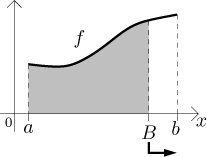If we cut away a part of the region along the right edge and make this cut-away part smaller and smaller, the resulting areas should converge to the whole area.

Similarly, f is Riemann integrable on [A,b], and in the second equality we cut away along the left edge.

The second property we will cover here is a modification of the standard Mean Value Theorem (see Derivatives - Theory - MVT) for the function F defined in the Fundamental Theorem of Calculus:The Fundamental theorem then says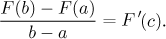When we substitute for F, we get the following thorem.

Theorem (The Mean Value Theorem for integrals, Lagrange theorem for integrals).
Let f be a continuous function on [a,b]. Then there is a number c in (a,b) such that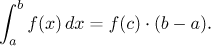If we recall the definition of an average of f (see Applications - Average), we can restate the Mean Value Theorem as follows:

Theorem.
Let f be a continuous function on [a,b]. Then there is a number c in (a,b) such that f (c) is equal to the average of f over the interval [a,b].

Note that the continuity in the assumption of the Mean Value Theorem is crucial. Indeed, recall the example of a jump function we saw before. Its average over [0,2] is 3/2, but the function is never equal to 3/2.

The Mean Value Theorem for integrals has many versions. The one we stated above is probably the most popular, but also the weakest, merely a reformulation of the good old MVT. There are much stronger statements, we will show one of the more popular here.

Theorem (The Mean Value Theorem for integrals).
Let f be a continuous function on [a,b] and g an integrable function on [a,b] that is positive there. Then there is a number c in (a,b) such that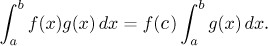Note that if you use this theorem with the constant function g(x) = 1, you get the first, weaker statement.